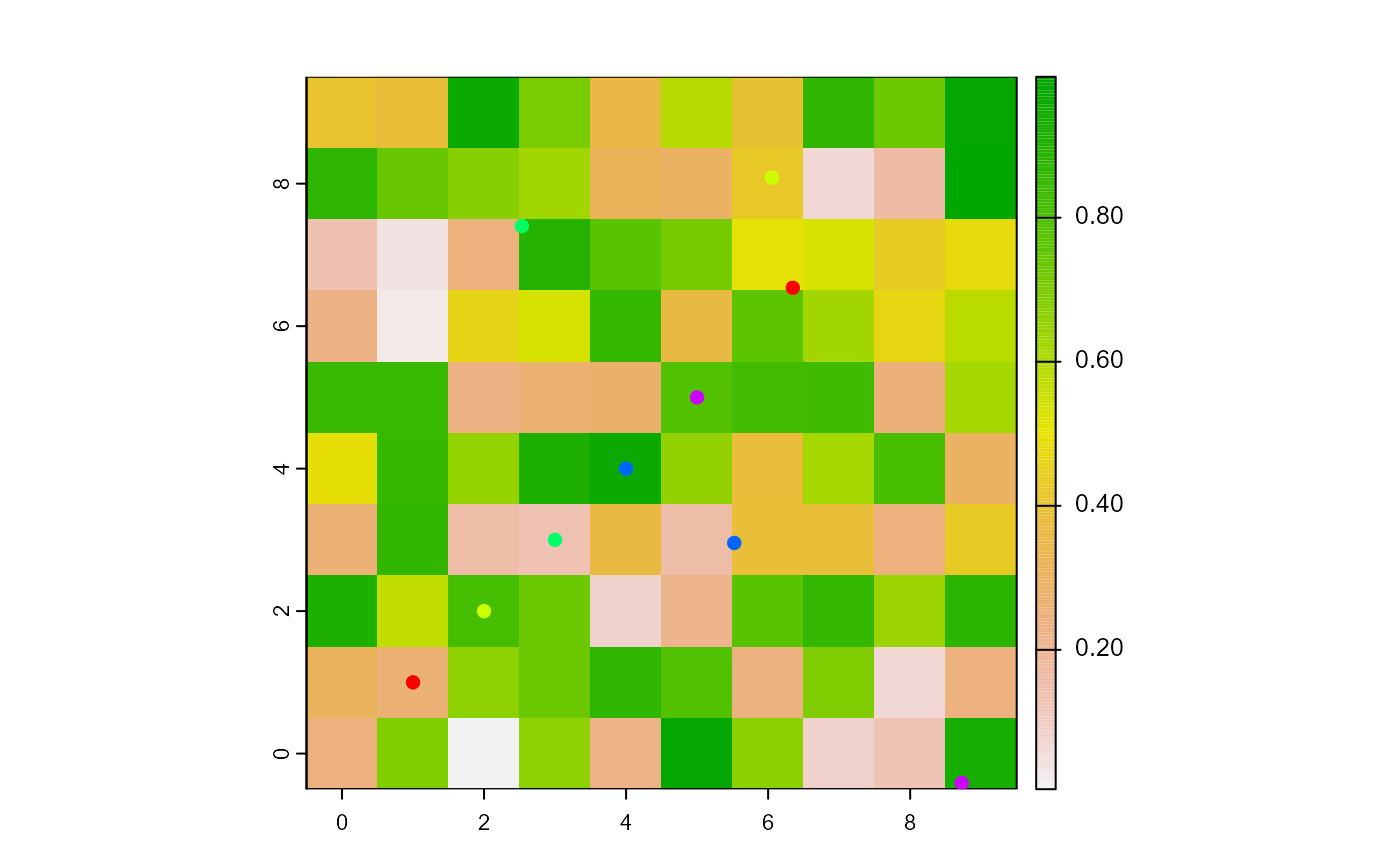Set the turtles xcor and ycor coordinates.

setXY(turtles, xcor, ycor, world, torus = FALSE)

# S4 method for agentMatrix,numeric,numeric,missing,ANY
setXY(turtles, xcor, ycor, torus)

# S4 method for agentMatrix,numeric,numeric,worldNLR,logical
setXY(turtles, xcor, ycor, world, torus = FALSE)

## Arguments

turtles

AgentMatrix object representing the moving agents.

xcor

Numeric. Vector of x coordinates. Must be of length 1 or of length turtles.

ycor

Numeric. Vector of y coordinates. Must be of length 1 or of length turtles.

world

WorldMatrix or worldArray object.

torus

Logical to determine if the world is wrapped. Default is torus = FALSE.

## Value

AgentMatrix representing the turtles with updated coordinates and updated data for their previous coordinates prevX and prevY.

## Details

world must be provided only if torus = TRUE.

     If the given coordinates [xcor, ycor]

are located outside of the world's extent and torus = TRUE,

then the coordinates assigned to the turtle

are the ones from a wrapped word; if torus = FALSE, the turtle

is located outside of the world's extent with the given coordinates.

Sarah Bauduin

## Examples

w1 <- createWorld(
minPxcor = 0, maxPxcor = 9, minPycor = 0, maxPycor = 9,
data = runif(100)
)
t1 <- createTurtles(n = 5, coords = randomXYcor(w1, n = 5))
plot(w1)
points(t1, col = of(agents = t1, var = "color"), pch = 16)

t1 <- setXY(turtles = t1, xcor = 1:5, ycor = 1:5)
points(t1, col = of(agents = t1, var = "color"), pch = 16)